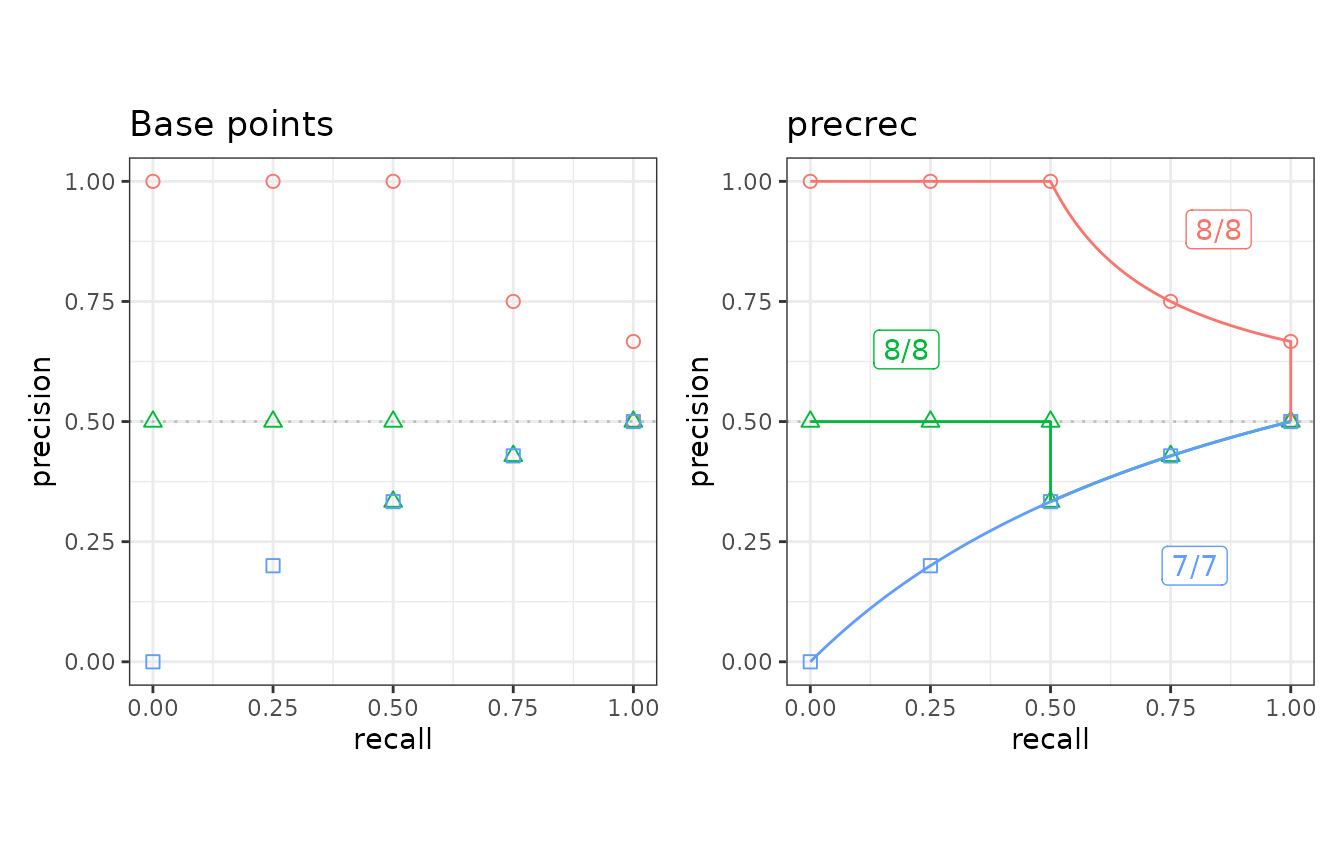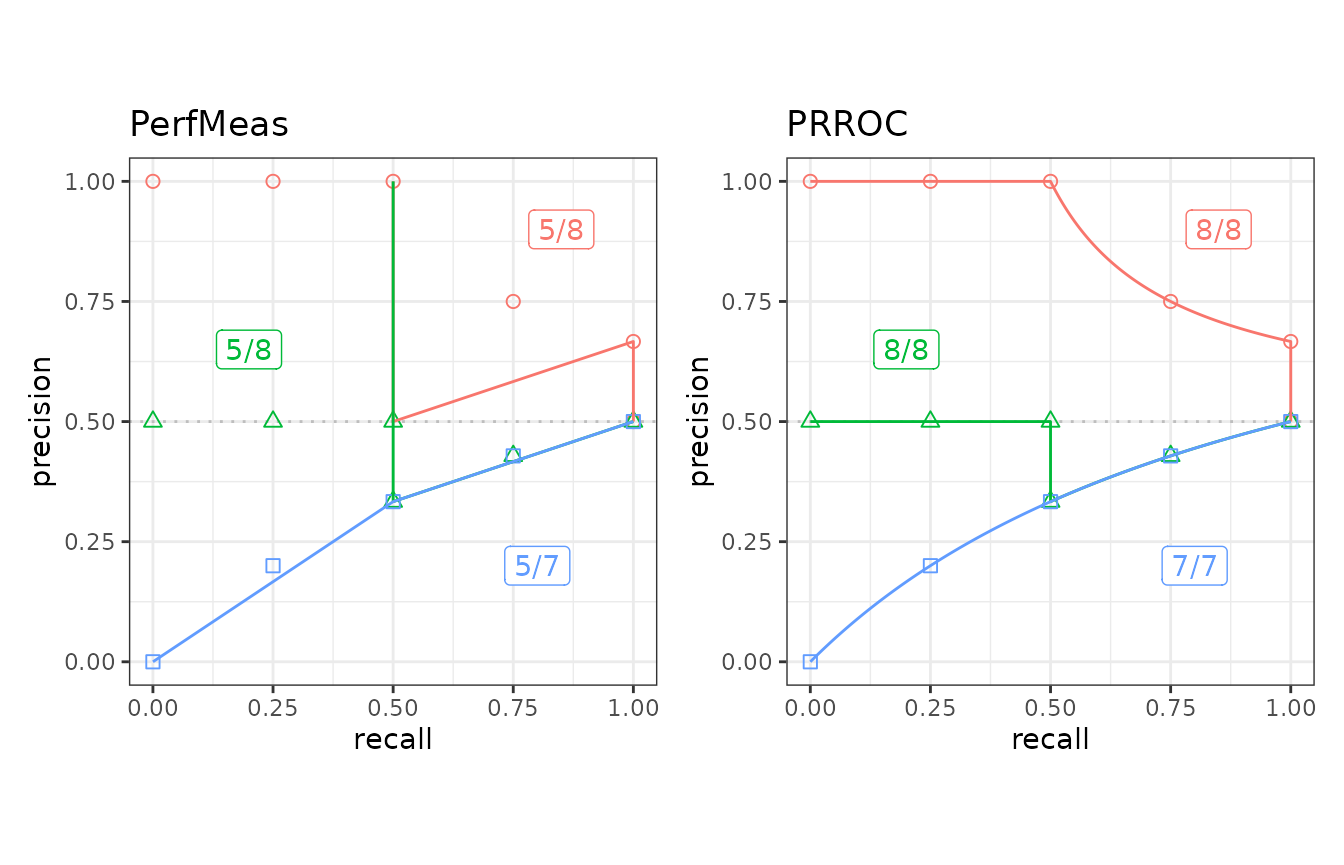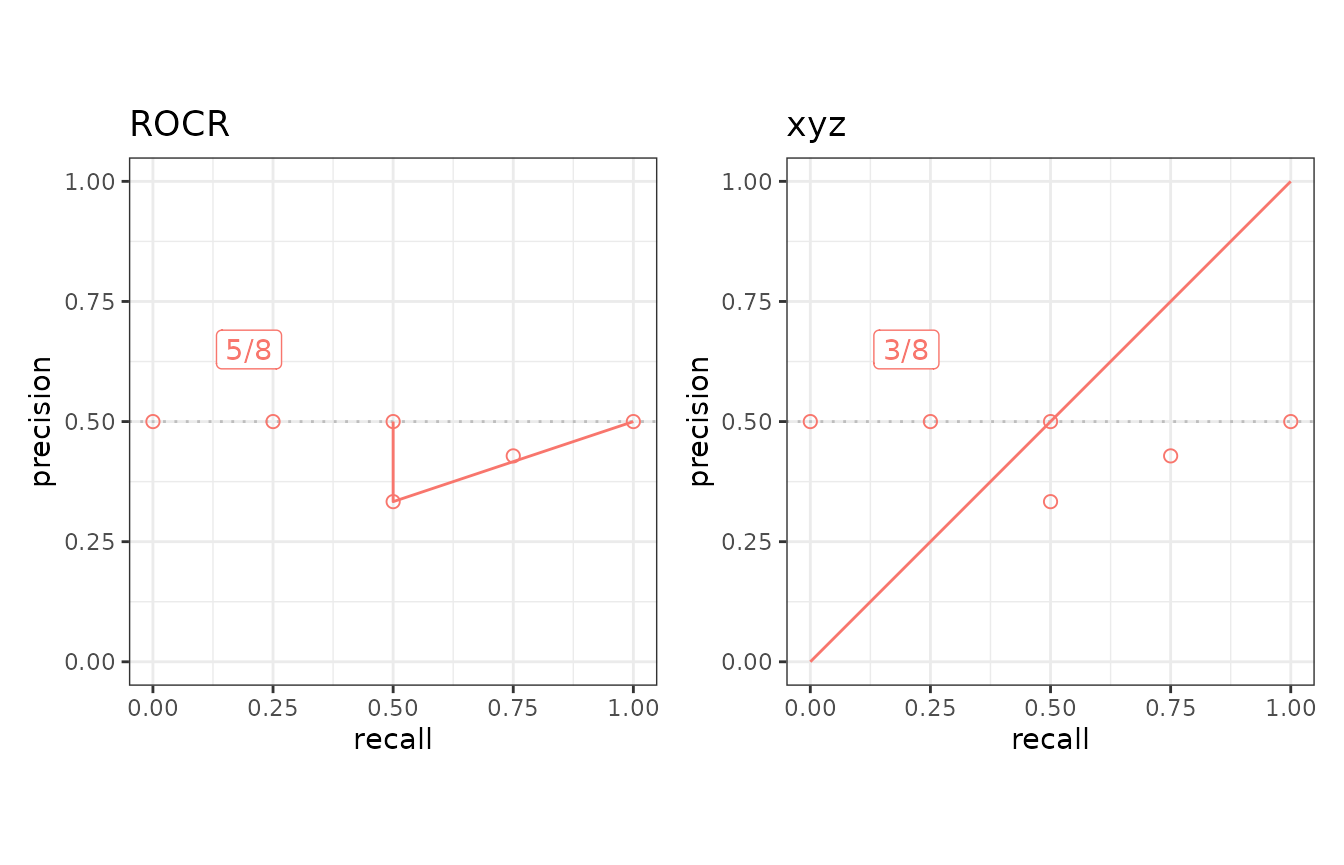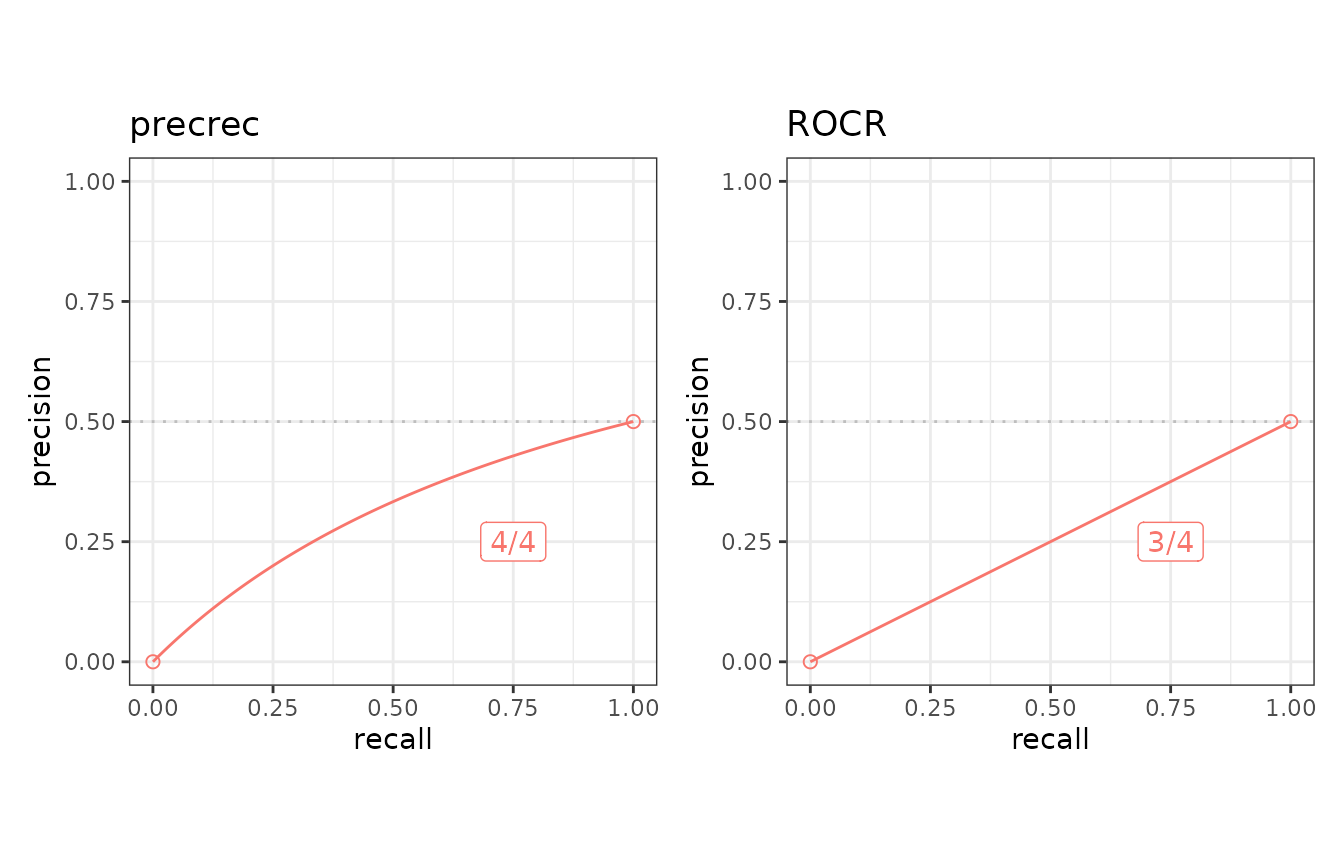The prcbench package is a testing workbench for evaluating precision-recall curves, which requires simple three step processes to perform evaluations of libraries that create precision-recall plots.

1. Tool selection by using the tool interface

2. Test data selection/creation by using the test data interface

1. Select pre-defined test data for the accuracy evaluation

2. Define randomly generated test data for the running-time evaluation

3. Run a evaluation function with the selected tools and test data sets

1. Accuracy evaluation of precision-recall curves

2. Running-time evaluation of precision-recall curves

In addition to predifined tools and test data sets, the prcbench package provides help functions for users to define their own tools and datasets.

1. User-defined tool interface

2. User-defined test data interface

1. User-defined test data for the accuracy evaluation

2. User-defined test data for the running-time evaluation

## 1. Tool interface

The prcbench package provides predefined interfaces for the following five tools that calculate precision-recall curves.

precrec R Tool web site, CRAN
ROCR R Tool web site, CRAN
PRROC R CRAN
AUCCalculator Java Tool web site
PerfMeas R CRAN

### Create a tool set

The create_toolset function generates a tool set with a combination of the five tools.

library(prcbench)

## A single tool
toolsetA <- create_toolset("ROCR")

## Multiple tools
toolsetB <- create_toolset(c("PerfMeas", "PRROC"))

## Tool sets can be manually combined to a single set
toolsetAB <- c(toolsetA, toolsetB)

### Arguments of the create_toolset function

The create_toolset function takes two additional arguments - calc_auc and store_res.

• calc_auc decides whether tools calculate AUC score or not (Calculation of AUCs are optional for the running-time evaluation, but not necessary for the evaluation of accurate precision-recall curves)

• store_res decides whether tools store the calculated curves or not (actual curves are required for the evaluation of accurate precision-recall curves)

### Use predefined tool sets

The following six tool sets are predefined with a different combination of tools along with default argument values.

Set name Tools calc_auc store_res
def5 ROCR, AUCCalculator, PerfMeas, PRROC, precrec TRUE TRUE
auc5 ROCR, xAUCCalculator, PerfMeas, PRROC, precrec TRUE FALSE
crv5 ROCR, AUCCalculator, PerfMeas, PRROC, precrec FALSE TRUE
def4 ROCR, AUCCalculator, PerfMeas, precrec TRUE TRUE
auc4 ROCR, AUCCalculator, PerfMeas, precrec TRUE FALSE
crv4 ROCR, AUCCalculator, PerfMeas, precrec FALSE TRUE
## Use 'set_names'
toolsetC <- create_toolset(set_names = "auc5")

## Multiple sets are automatically combined to a single set
toolsetD <- create_toolset(set_names = c("auc5", "crv4"))

## 2. Test data interface

The prcbench package provides two different types of test data sets.

1. curve: evaluates the accuracy of precision-recall curves
2. bench: measures running times of creating precision-recall curves

The create_testset function offers both types of test data by setting the first argument either as “curve” or “bench”.

### 2a. Select pre-defined test data for the accuracy evaluation

The create_testset function takes predefined set names for curve evaluation. These data sets contain pre-calculated precision and recall values. The pre-calculated values must be correct so that they can be compared with the results of specified tools.

The following four test sets are currently available.

name #scores&labels #pos labels #neg labels expected #points expected start expected end
c1 4 2 2 6 (0, 1) (1, 0.5)
c2 4 2 2 6 (0, 0.5) (1, 0.5)
c3 4 2 2 6 (0, 0) (1, 0.5)
c4 8 4 4 9 (0, 1) (1, 0.5)
## C1 test set
testset2A <- create_testset("curve", "c1")

## C2 test set
testset2B <- create_testset("curve", "c2")

## Test data sets can be manually combined to a single set
testset2AB <- c(testset2A, testset2B)

## Multiple sets are automatically combined to a single set
testset2C <- create_testset("curve", c("c1", "c2"))

### 2b. Create randomly generated test data for the running-time evaluation

The create_testset function uses a naming convention for randomly generated data for benchmarking. The format is a prefix (‘b’ or ‘i’) followed by the number of dataset. The prefix ‘b’ indicates a balanced dataset, whereas ‘i’ indicates an imbalanced dataset. The number can be used with a suffix ‘k’ or ‘m’, indicating respectively 1000 or 1 million.

## A balanced data set with 50 positives and 50 negatives
testset1A <- create_testset("bench", "b100")

## An imbalanced data set with 2500 positives and 7500 negatives
testset1B <- create_testset("bench", "i10k")

## Test data sets can be manually combined to a single set
testset1AB <- c(testset1A, testset1B)

## Multiple sets are automatically combined to a single set
testset1C <- create_testset("bench", c("i10", "b10"))

## 3. Run a evaluation function with the selected tools and test data sets

The prcbench package currently provides two differnt types of peformance evaluation.

1. Accuracy evaluation of precision-recall curves

2. Running-time evaluation of precision-recall curves

### 3a. Accuracy evaluation of precision-recall curves

The run_evalcurve function evaluates precision-recall curves with the following five test cases. The basic idea is that the function returns the full score as long as the points generated by a library matches with the manually calculated recall and precision values.

Test case Description
fpoint Check the first point
int_pts Check the intermediate points
epoint Check the end point
x_range Evaluate a range between two recall values
y_range Evaluate a range between two precision values

#### Evaluation scores

The run_evalcurve function calculates the scores of the test cases and summarizes them to a data frame.

## Evaluate precision-recall curves for ROCR and precrec with c1 test set
testset <- create_testset("curve", "c1")
toolset <- create_toolset(c("ROCR", "precrec"))
scores <- run_evalcurve(testset, toolset)
scores
##   testset toolset toolname score
## 1      c1 precrec  precrec   8/8
## 2      c1    ROCR     ROCR   5/8

The result of each test case can be displayed by specifying data_type = all of the print function.

## Print all results
print(scores, data_type = "all")
##    testset toolset toolname testitem testcat success total
## 1       c1 precrec  precrec  x_range      Rg       1     1
## 2       c1 precrec  precrec  y_range      Rg       1     1
## 3       c1 precrec  precrec   fpoint      SE       1     1
## 4       c1 precrec  precrec   intpts      Ip       4     4
## 5       c1 precrec  precrec   epoint      SE       1     1
## 6       c1    ROCR     ROCR  x_range      Rg       1     1
## 7       c1    ROCR     ROCR  y_range      Rg       1     1
## 8       c1    ROCR     ROCR   fpoint      SE       0     1
## 9       c1    ROCR     ROCR   intpts      Ip       2     4
## 10      c1    ROCR     ROCR   epoint      SE       1     1

#### Visualization of the result

The autoplot shows a plot with the result of the run_evalcurve function.

## ggplot2 is necessary to use autoplot
library(ggplot2)

## Plot base points and the result of precrec on c1, c2, and c3 test sets
testset <- create_testset("curve", c("c1", "c2", "c3"))
toolset <- create_toolset("precrec")
scores1 <- run_evalcurve(testset, toolset)
autoplot(scores1)

## Plot the results of PerfMeas and PRROC on c1, c2, and c3 test sets
toolset <- create_toolset(c("PerfMeas", "PRROC"))
scores2 <- run_evalcurve(testset, toolset)
autoplot(scores2, base_plot = FALSE)### 3b. Running-time evaluation of precision-recall curves

The run_benchmark function internally calls the microbenchmark function provided by the microbenchmark package. It takes a test set and a tool set and returns the result of microbenchmark.

## Run microbenchmark for aut5 on b10
testset <- create_testset("bench", "b10")
toolset <- create_toolset(set_names = "auc5")
res <- run_benchmark(testset, toolset)
res
##   testset toolset      toolname   min    lq  mean median    uq   max neval
## 1     b10    auc5 AUCCalculator 1.972 2.776 7.166   2.95 3.625 24.50     5
## 2     b10    auc5      PerfMeas 0.064 0.066 0.099   0.07 0.087  0.21     5
## 3     b10    auc5       precrec 4.441 4.458 4.581   4.51 4.538  4.96     5
## 4     b10    auc5         PRROC 0.157 0.161 0.199   0.17 0.190  0.32     5
## 5     b10    auc5          ROCR 2.009 2.029 2.100   2.03 2.085  2.35     5

## 4. Create a user-defined tool

In addition to the predefined five tools, users can add new tool interfaces for their own tools to run benchmarking and curve evaluation. The create_usrtool function takes a name of the tool and a function for calculating a precision-recall curve.

## Create a new tool set for 'xyz'
toolname <- "xyz"
calcfunc <- create_example_func()
toolsetU <- create_usrtool(toolname, calcfunc)

## User-defined tools can be combined with predefined tools
toolsetA <- create_toolset("ROCR")
toolsetU2 <- c(toolsetA, toolsetU)

Like the predefined tool sets, user-defined tool sets can be used for both run_benchmark and run_evalcurve.

## Curve evaluation
testset3 <- create_testset("curve", "c2")
scores3 <- run_evalcurve(testset3, toolsetU2)
autoplot(scores3, base_plot = FALSE)### The format of the function for calculating a precision-recall curve

The create_example_func function creates an example for the second argument of the create_usrtool function. The actual function should also take a testset generated by the create_testset function and returns a list with three elements - x, y, and auc.

## Show an example of the second argument
calcfunc <- create_example_func()
print(calcfunc)
## function (single_testset)
## {
##     scores <- single_testset\$get_scores()
##     list(x = seq(0, 1, 1/length(scores)), y = seq(0, 1, 1/length(scores)),
##         auc = 0.5)
## }
## <bytecode: 0x564cc15f5668>
## <environment: 0x564cc06e3180>

The create_testset function produces a testset as either TestDataB or TestDataC object. See the help files of the R6 classes - help(TestDataB) and help(TestDataC) - for the methods that can be used with the precision-recall calculation.

## 5. Create a user-defined test data

The prcbench package also supports user-defined test data interfaces. The create_usrdata function creates two types of test datasets.

1. User-defined test data for the accuracy evaluation

2. User-defined test data for the running-time evaluation

### 5a. User-defined test data for the accuracy evaluation

The first argument of the create_usrdata function should be “curve” to create a test dataset for the accuracy evaluation. Scores and labels as well as pre-calculated recall and precision values are required. These pre-calculated values are used to compare with the corresponding values predicted by the specified tools.

## Create a test dataset 'c5' for benchmarking
testsetC <- create_usrdata("curve",
scores = c(0.1, 0.2), labels = c(1, 0),
tsname = "c5", base_x = c(0.0, 1.0),
base_y = c(0.0, 0.5)
)

It can be used in the same way as the predefined test datasets selected by create_testset.

## Run curve evaluation for ROCR and precrec on a predefined test dataset
toolset2 <- create_toolset(c("ROCR", "precrec"))
scores2 <- run_evalcurve(testsetC, toolset2)
autoplot(scores2, base_plot = FALSE)#### 5b. User-defined test data for the running-time evaluation

The first argument of the create_usrdata function should be “bench” to create a test dataset for the running-time evaluation. Scores and labels are also required.

## Create a test dataset 'b5' for benchmarking
testsetB <- create_usrdata("bench",
scores = c(0.1, 0.2), labels = c(1, 0),
tsname = "b5"
)

It can be used in the same way as the test datasets generated by create_testset.

## Run microbenchmark for ROCR and precrec on a predefined test dataset
toolset <- create_toolset(c("ROCR", "precrec"))
res <- run_benchmark(testsetB, toolset)
res
##   testset toolset toolname min  lq mean median  uq max neval
## 1      b5 precrec  precrec 4.4 4.5  4.6    4.5 4.5 5.0     5
## 2      b5    ROCR     ROCR 2.0 2.0  2.1    2.1 2.1 2.3     5

See our website - Classifier evaluation with imbalanced datasets – for useful tips for performance evaluation on binary classifiers. In addition, we have summarized potential pitfalls of ROC plots with imbalanced datasets. See our paper – The Precision-Recall Plot Is More Informative than the ROC Plot When Evaluating Binary Classifiers on Imbalanced Datasets - for more details.